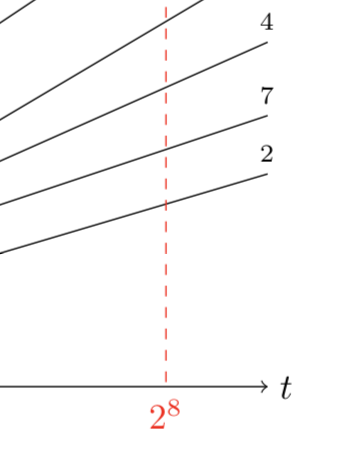Collatz meets Fibonacci

To appear in The MAA Mathematics MagazineThe Collatz map is defined for a positive even integer as half that integer, and for a positive odd integer as that integer threefold, plus one. The Collatz conjecture states that when the map is iterated the number one is eventually reached. We study permutations that arise as sequences from this iteration. We show that permutations of this type of length up to 14 are enumerated by the Fibonacci numbers. Beyond that excess permutations appear. We will explain the appearance of these excess permutations and give an upper bound on the exact enumeration.